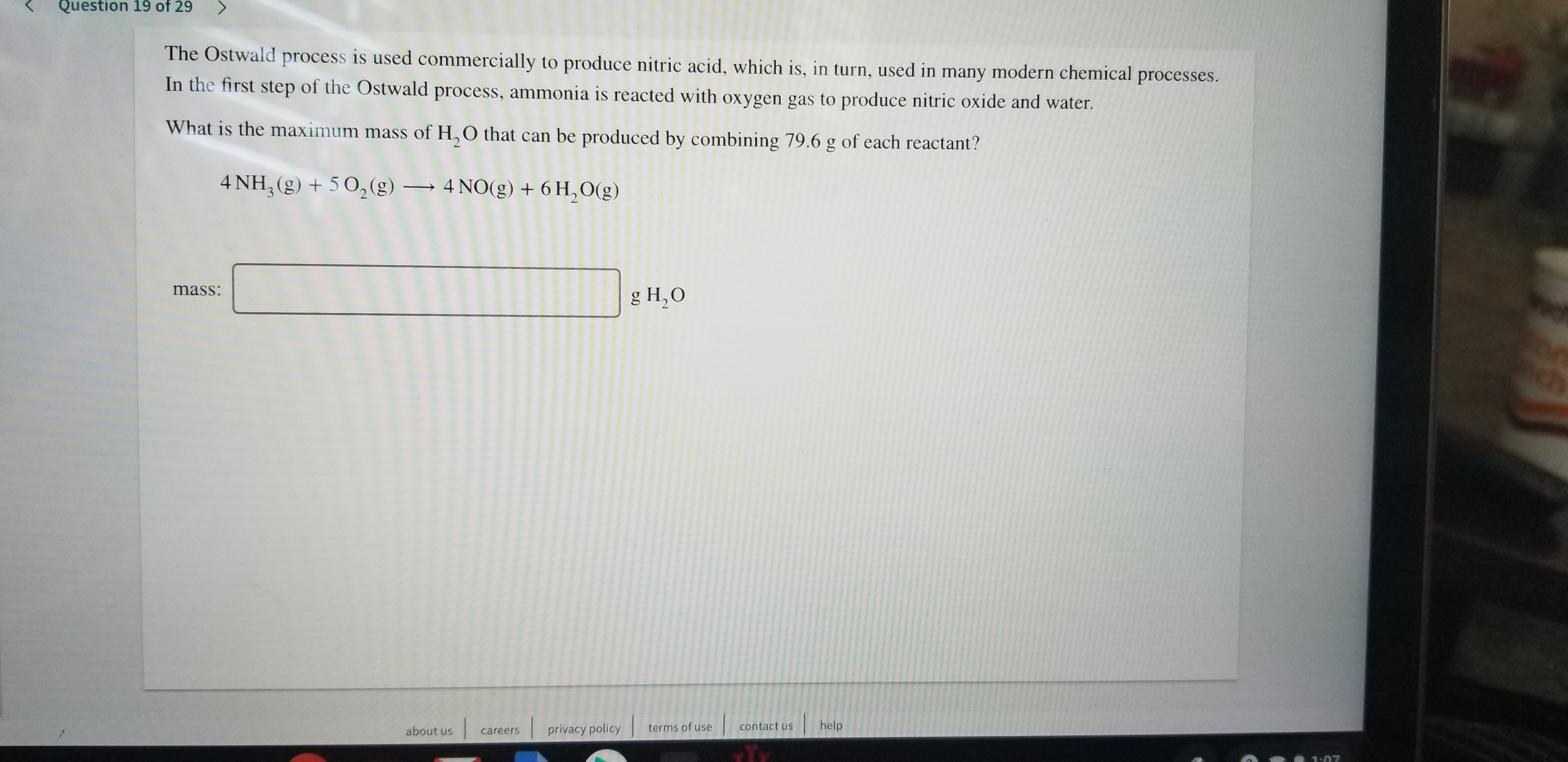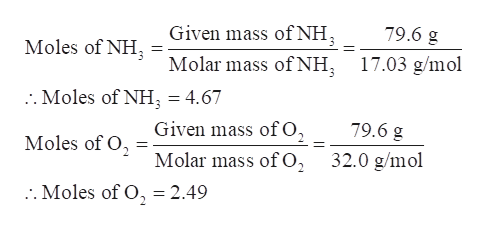# Question 19 of 29The Ostwald process is used commercially to produce nitric acid, which is, in turn, used in many modern chemical processes.In the first step of the Ostwald process, ammonia is reacted with oxygen gas to produce nitric oxide and water.What is the maximum mass of H,O that can be produced by combining 79.6 g of each reactant?4 NH, (g)502(g)4 NO(g) + 6H,O(g)mass:g H2Ohelpprivacy policycontact usterms of useabout uscareers1:07

Question
308 viewshelp_outlineImage TranscriptioncloseQuestion 19 of 29 The Ostwald process is used commercially to produce nitric acid, which is, in turn, used in many modern chemical processes. In the first step of the Ostwald process, ammonia is reacted with oxygen gas to produce nitric oxide and water. What is the maximum mass of H,O that can be produced by combining 79.6 g of each reactant? 4 NH, (g)502(g) 4 NO(g) + 6H,O(g) mass: g H2O help privacy policy contact us terms of use about us careers 1:07 fullscreen
check_circle

Step 1

The Ostwald process is used commercially to produce nitric acid, which is, in turn, used in many modern chemical processes. In the first step of the Ostwald process, ammonia is reacted with oxygen gas to produce nitric oxide and water. The maximum mass of H2O that can be produced by combining 79.6 g of each reactant is to be calculated.

Step 2

Calculate the moles of ammonia and oxygen gas-

Given mass of NH3 = 79.6 g

Molar mass of NH3 = 17.03 g/mol

Given mass of O2 = 79.6 g

Molar mass of O2 = 32.0 g/molhelp_outlineImage Transcriptionclose79.6 g Given mass of NH Moles of NH 17.03 g/mol Molar mass of NH . Moles of NH = 4.67 79.6 g Given mass of O, Moles of O2 32.0 g/mol Molar mass of O . Moles of O, = 2.49 fullscreen
Step 3

Determine the limiting reagent –

As per the balanced equation, 4 moles of ammonia react...help_outlineImage Transcriptionclose5 moles of O, reacts with 4 moles of NH3 4 moles of NH 5 moles of O :. 2.49 moles of O2 will react with (2.49 moles O2) x = 1.99 moles NH fullscreen

### Want to see the full answer?

See Solution

#### Want to see this answer and more?

Solutions are written by subject experts who are available 24/7. Questions are typically answered within 1 hour.*

See Solution
*Response times may vary by subject and question.
Tagged in

### General Chemistry